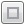You have the option of entering or changing any part of a formula in text mode. Text mode displays the formula in JMP Scripting Language (JSL). The entire formula (or any of its terms) appears in the Script Editor when you double-click the formula. If the formula is long, it opens in a maximized Script Editor window. Otherwise, you can click the Maximize editor buttonto maximize the window.
Any element of a formula can be displayed in the Script Editor and then edited. After editing formula scripting commands, click outside the formula (or click OK below the maximized window) to see its formatted form and to save the change. To enter an If statement in text form, add pairs of arguments for each If/Then clause in the statement, and a single last argument for the else clause if needed. In text form, the If statement in Figure 6.17 looks like this: If(:total!=0, (:count/:total)*100, 0).
Figure 6.17 An If Statement in Formula Mode

Help created on 7/12/2018